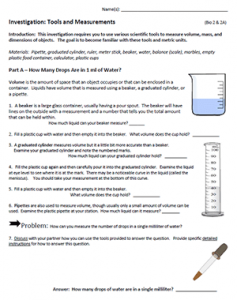# Investigation: Tools and MeasurementsThis activity is designed to help students become familiar with basic equipment found in the lab.  Students are asked to take volume measurements using a graduated cylinder, beaker, and a 3ml pipette.  Ultimately, they must determine how many single drops of water are in 1 milliliter by designing a way to test this given the tools provided.   They can either use the 3ml pipette or the graduated cylinder to answer the question.

Students then examine how volume is calculated using a square container  (length x width x height) and then comparing that to the amount of fluid can be contained within it by using a beaker.   They will need to develop an explanation for why the numbers are not the same (different units.) Students will also use a water displacement technique to determine the volume of a marble by filling the cylinder to 20 ml, then dropping three marbles into it to see how much the water rises.

Students also learn how to use a balance to determine mass and use a meter stick to measure the heights of objects in the lab.  Most students will know how to do these things, but it serves as a good reminder to students to always use the metric system in science.  I no longer do conversions with students and instead teach them to take measurements using proper units.